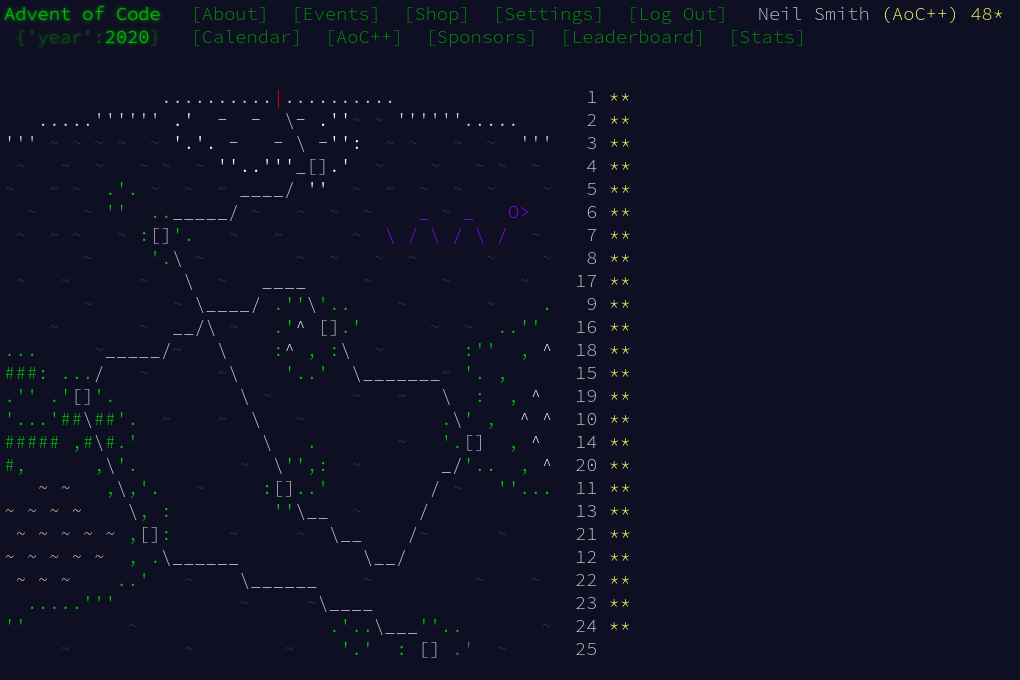January 9, 2021

# Advent of Code 2020 day 24

More cellular automata, but with added typeclass funThe solution to day 24 was very similar to my solution to day 17 and most of the code for today is much the same as then.

## Part 1

Parsing and data structures were the bulk of the work for part 1. I thought about using the hexGrid library, but decided that I wouldn't take too much advantage of it. Instead I used the same coordinate system and implemented it myself. East-west rows are constant y, and rows from south-west to north-east are constant x. Moving south-east or north-west means changing both x and y.A Tile is defined by its location, given as a V2 two-dimensional vector, and a Grid is a Set of black tiles. There are six Directions, and the function delta converts a Direction into a change in position. Finally, I fix how the elements of a Tile combine to make parsing a bit easier.

data Direction = NE | E | SE | SW | W | NW
deriving (Show, Eq, Enum, Bounded)

type Tile = V2 Int -- x, y
type Grid = S.Set Tile

instance Semigroup Int where
(<>) = (+)

instance Monoid Int where
mempty = 0

delta :: Direction -> Tile
delta NE = V2  1  0
delta E  = V2  0  1
delta SE = V2 -1  1
delta SW = V2 -1  0
delta W  = V2  0 -1
delta NW = V2  1 -1


Parsing the input file has a couple of subtleties. One is the ordering of elements in the choice, so that "n" and "s" characters are consumed before "e" and "w". The other is the use of the semigroup and monoid instances to allow foldMap to combine all the steps into the position at the end of each path.

tilesP = tileP sepBy endOfLine
tileP = foldMap delta <$> many1 stepP stepP = choice [neP, nwP, seP, swP, eP, wP] neP = "ne" *> pure NE nwP = "nw" *> pure NW seP = "se" *> pure SE swP = "sw" *> pure SW eP = "e" *> pure E wP = "w" *> pure W  All that's left is to create the grid by flipping tiles, then count how many are black. main :: IO () main = do text <- TIO.readFile "data/advent24.txt" let walks = successfulParse text let grid0 = foldr flipTile S.empty walks print$ part1 grid0

part1 grid0 = S.size grid0

flipTile :: Tile -> Grid -> Grid
flipTile tile tiles
| tile S.member tiles = S.delete tile tiles
| otherwise = S.insert tile tiles


## Part 2

This is very similar to day 17. As I defined the directions as both Enum and Bounded, I can generate all the neighbours of a point by enumerating all the directions.

neighbourSpaces :: Tile -> Grid
neighbourSpaces here = S.fromList $map nbrSpace [minBound .. maxBound] -- [NE .. NW] where nbrSpace d = here ^+^ (delta d)  After that, it's all much the same as before, but with the grid updating by adding newly black tiles and removing newly white tiles. part2 grid0 = S.size$ (iterate update  grid0) !! 100

countOccupiedNeighbours :: Tile -> Grid -> Int
countOccupiedNeighbours cell grid =
S.size $S.intersection grid$ neighbourSpaces cell

tileBecomesWhite :: Grid -> Tile -> Bool
tileBecomesWhite grid cell = black && ((nNbrs == 0) || (nNbrs > 2))
where black = cell S.member grid
nNbrs = countOccupiedNeighbours cell grid

tileBecomesBlack :: Grid -> Tile -> Bool
tileBecomesBlack grid cell = white && (nNbrs == 2)
where white = cell S.notMember grid
nNbrs = countOccupiedNeighbours cell grid

update :: Grid -> Grid
update grid = (grid S.union newBlacks) S.difference newWhites
where neighbours = (S.foldr mergeNeighbours S.empty grid) S.difference grid
mergeNeighbours cell acc = S.union acc \$ neighbourSpaces cell
newWhites = S.filter (tileBecomesWhite grid) grid
newBlacks = S.filter (tileBecomesBlack grid) neighbours


## Code

You can find the code here or on GitLab.